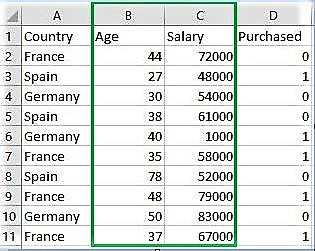# sklearn.Binarizer() in Python

• Last Updated : 29 Jun, 2021

sklearn.preprocessing.Binarizer() is a method which belongs to preprocessing module. It plays a key role in the discretization of continuous feature values.
Example #1:
A continuous data of pixels values of an 8-bit grayscale image have values ranging between 0 (black) and 255 (white) and one needs it to be black and white. So, using Binarizer() one can set a threshold converting pixel values from 0 – 127 to 0 and 128 – 255 as 1.
Example #2:
One has a machine record having “Success Percentage” as a feature. These values are continuous ranging from 10% to 99% but a researcher simply wants to use this data for prediction of pass or fail status for the machine based on other given parameters.
Syntax :

`sklearn.preprocessing.Binarizer(threshold, copy)`

Parameters :
threshold :[float, optional] Values less than or equal to threshold is mapped to 0, else to 1. By default threshold value is 0.0.
copy :[boolean, optional] If set to False, it avoids a copy. By default it is True.

Return :

`Binarized Feature values`Below is the Python code explaining sklearn.Binarizer()

## Python3

 `# Python code explaining how` `# to Binarize feature values` ` `  `""" PART 1` `    ``Importing Libraries """` ` `  `import` `numpy as np` `import` `matplotlib.pyplot as plt` `import` `pandas as pd`   `# Sklearn library ` `from` `sklearn ``import` `preprocessing`   `""" PART 2` `    ``Importing Data """` ` `  `data_set ``=` `pd.read_csv(` `        ``'C:\\Users\\dell\\Desktop\\Data_for_Feature_Scaling.csv'``)` `data_set.head()`   `# here Features - Age and Salary columns ` `# are taken using slicing` `# to binarize values` `age ``=` `data_set.iloc[:, ``1``].values` `salary ``=` `data_set.iloc[:, ``2``].values` `print` `(``"\nOriginal age data values : \n"``,  age)` `print` `(``"\nOriginal salary data values : \n"``,  salary)`   `""" PART 4` `    ``Binarizing values """`   `from` `sklearn.preprocessing ``import` `Binarizer`   `x ``=` `age` `x ``=` `x.reshape(``1``, ``-``1``)` `y ``=` `salary` `y ``=` `y.reshape(``1``, ``-``1``)`   `# For age, let threshold be 35` `# For salary, let threshold be 61000` `binarizer_1 ``=` `Binarizer(``35``)` `binarizer_2 ``=` `Binarizer(``61000``)`   `# Transformed feature` `print` `(``"\nBinarized age : \n"``, binarizer_1.fit_transform(x))`   `print` `(``"\nBinarized salary : \n"``, binarizer_2.fit_transform(y))`

Output :

```   Country  Age  Salary  Purchased
0   France   44   72000          0
1    Spain   27   48000          1
2  Germany   30   54000          0
3    Spain   38   61000          0
4  Germany   40    1000          1

Original age data values :
[44 27 30 38 40 35 78 48 50 37]

Original salary data values :
[72000 48000 54000 61000  1000 58000 52000 79000 83000 67000]

Binarized age :
[[1 0 0 1 1 0 1 1 1 1]]

Binarized salary :
[[1 0 0 0 0 0 0 1 1 1]]```

My Personal Notes arrow_drop_up
Recommended Articles
Page :